# Problem 52589. Circular Segment Area

Let us consider a circle with radius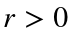. If we draw an angle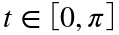(in radians) from the center of the circle, the two radii forming that angle intersect the circle at A and B. We call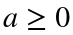the area delimited by the chord and the arc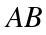(the so-called Circular Segment, blu in the figure).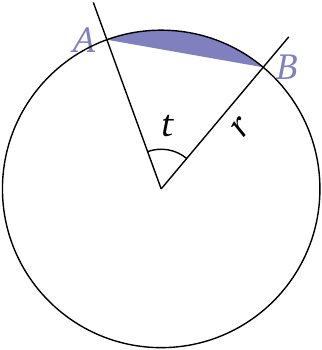For a given radius r and area a, compute the angle t (rounded to 6 significant digits).

### Solution Stats

40.74% Correct | 59.26% Incorrect
Last Solution submitted on Aug 10, 2023

### Community Treasure Hunt

Find the treasures in MATLAB Central and discover how the community can help you!

Start Hunting!